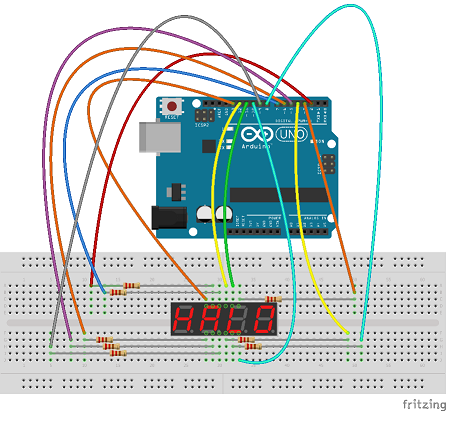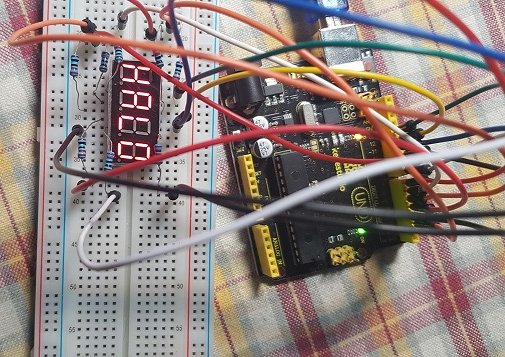# B4R TutorialSevSeg with inline C

This B4R project uses the SevSeg library via inline C to display characters on a 4 digit x 7 segment LED display (I am using a SMA420364L) - hard wired.

The inline C code via the SevSeg library does all the heavy lifting to display the chars on the 4 digit display. It can be used for common Anode and common Cathode displays as well as 1 to 4 digit displays. I have only tested in on a common Cathode display with 4 digits. The is an abundance of comments in the B4R code that explains setting the mode, number of digits, pins that will be used (see below wiring diagram), position of where the decimal point should be displayed, and brightness of the display (brightness from 0 to 100)

The attached B4R project simulates a clock. It displays the Hours:Minutes, then the Minutes:Seconds, and then just "HALO" and then cycles through the same sequence again.

Extract SevSeg.zip and copy the folder (with the .h and .cpp files) to your arduino libs folder.

Edit: Have posted a wrapper for SevSeg hereSample Code:

B4X:
``````#Region Project Attributes
#AutoFlushLogs: True
#CheckArrayBounds: True
#StackBufferSize: 300
#End Region

Sub Process_Globals
'These global variables will be declared once when the application starts.
'Public variables can be accessed from all modules.
Public Serial1 As Serial

'    Dim bc As ByteConverter

Dim mode_in As Boolean = False                    'common cathode (set to True for display with common Anode)
Dim numOfDigits As Byte = 4                       'Using a 4 digit x 7 segment LED display
Dim digit1 As Byte = 13                           'Most significant digit i.e most left - pin 13
Dim digit2 As Byte = 12                           '2nd digit from left - pin 12
Dim digit3 As Byte = 11                           '3rd digit from left - pin 11
Dim digit4 As Byte = 10                           'Least significant digit i.e most right - pin 10
Dim segmentDP As Byte = 9                         'digital point - pin 9
Dim segment1 As Byte = 2                          'Segment A - pin 2
Dim segment2 As Byte = 3                          'Segment B - pin 3
Dim segment3 As Byte = 4                          'Segment C - pin 4
Dim segment4 As Byte = 5                          'Segment D - pin 5
Dim segment5 As Byte = 6                          'Segment E - pin 6
Dim segment6 As Byte = 7                          'Segment F - pin 7
Dim segment7 As Byte = 8                          'Segment G - pin 8
Dim brightness As Byte = 100                      'Set the brightness of the LED display between 0 and 100

Dim min1, min10, hr1, hr10, sec10, sec1 As Byte   'keeping track of out clock thta we are setting up in AppStart
Dim decimalposition As Byte = 2                   'set the decimal position to 2 (the 2nd DP from the left)
Dim prevtime, currenttime As ULong

Dim dat1() As Byte = "HALO"                       'set dat1 to "abcd" - we will pass it to the dsipaly in the timer

Dim cnt As UInt = 0                               'a counter to decide what to display
'display will chnage between showing hour and minutes, minutes and seconds, "abcd", and then repeating this sequence of display
Dim t As Timer                                    'We need a timer

End Sub

Private Sub AppStart
Serial1.Initialize(115200)
Log("AppStart")
t.Initialize("t_tick", 20)                        'initialize the timer - tick every 20ms
prevtime = Millis                                 'get the millis since MCU started

hr10 = 1                                          'Set the hours, minuntes, and seconds here that you want to start the clock with
hr1 = 9
min10 = 2
min1 = 2
sec10 = 0
sec1 = 0

RunNative("begin", Null)                          'call Begin in SevSeg
Delay(1)
RunNative("SetBrightness", Null)                  'set the brightness of the LED's
Delay(1)
t.Enabled = True                                  'Enable the timer

End Sub

Sub t_tick

cnt = cnt + 1                                     'increment the counter

currenttime = Millis                              'get the current Millis
If (currenttime - prevtime) > 1000 Then           'has 1 second gone by since previous "get Millis"?
prevtime = currenttime                        'if so, then store current time to previous time
sec1 = sec1 + 1                               'increment seconds 1's
If sec1 > 9 Then
sec1 = 0
sec10 = sec10 + 1                         'increment seconds 10's
If sec10 > 5 Then                         '.....sure you will follow the rest of the logic
sec10 = 0
min1 = min1 + 1
If min1 > 9 Then
min1 = 0
min10 = min10 + 1
If min10 > 5 Then
min10 = 0
hr1 = hr1 + 1
If hr1 > 9 Then
hr1 = 0
hr10 = hr10 + 1
End If
End If
End If
End If
End If
End If

If hr10 = 2 Then                             'check for midnight and if so set hr10 and hr1 to 0
If hr1 = 4 Then
hr10 = 0
hr1 = 0
End If
End If

If cnt < 500 Then                                                            'if counter is less than 500 then display HH.MM
decimalposition = 2
Dim c As String = JoinStrings(Array As String(hr10, hr1, min10, min1))   'build the string
Dim b() As Byte = c                                                      'convert the string to a byte array
RunNative("DisplayString", b)                                            'call DisplayString in SevSeg and pass it the byte array
else if cnt < 1000 Then                                                      'if counter is > 500 and less than 1000 then display MM.SS
decimalposition = 2
Dim c As String = JoinStrings(Array As String(min10, min1, sec10, sec1))
Dim b() As Byte = c
RunNative("DisplayString", b)
else if cnt < 1500 Then                                                      'if counter >= 1000 but < 1500 then display dat1 = abcd
decimalposition = 0
RunNative("DisplayString", dat1)
Else
cnt = 0
End If

End Sub

#if C

#include <SevSeg.h>

SevSeg sevseg;                                                                 //We are using the SevSeg library

void begin(B4R::Object* o) {                                                   //begin - called from B4R code to set up mode(common cathode/anode), number of digits on the display, pins that are used, and where the digital point should be displayed
sevseg.Begin(b4r_main::_mode_in, b4r_main::_numofdigits, b4r_main::_digit1, b4r_main::_digit2, b4r_main::_digit3, b4r_main::_digit4, b4r_main::_segment1, b4r_main::_segment2, b4r_main::_segment3, b4r_main::_segment4, b4r_main::_segment5, b4r_main::_segment6, b4r_main::_segment7, b4r_main::_segmentdp);
}

void SetBrightness(B4R::Object* o) {                                           //set the brightness of the LED's on the 7 segment display
sevseg.SetBrightness(b4r_main::_brightness);
}

void DisplayString(B4R::Object* o) {                                          //what do display - passed in from the B4R code and received here as a B4R Object
B4R::Array* conversion = (B4R::Array*)B4R::Object::toPointer(o);
char* toDisplay = (char*)conversion->data;
sevseg.DisplayString(toDisplay, b4r_main::_decimalposition);
}

#End If``````#### Attachments

• SevSeg.zip
5.5 KB · Views: 200
• b4rSevSegInline.zip
2.5 KB · Views: 178
Last edited:

Replies
1
Views
353
B4R Library rSevSeg
Replies
0
Views
1K
Replies
18
Views
6K
Replies
8
Views
6K
Replies
2
Views
3K